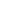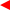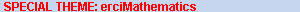contentsERCIM News No.50, July 2002# Mathematics on the Web with MathML

by Max Froumentin

MathML 2.0 has become a W3C Recommendation in February 2001 and is now widely supported, making it possible at last to distribute scientific material on the Web.

Although mathematical notation has made its way into computer-generated documents with quality publishing software now correctly handling mathematics, putting mathematics on the Web has been a problem since the beginning of HTML since support for displaying mathematics was minimal. Shortly after the birth of XML, the W3C started designing MathML: an XML language for expressing mathematics, in order to make it possible to display formulas in Web browsers as well as provide a interchange format for mathematical software.

Mathematics and the Web
Mathematical notation inherits from centuries of refinement, resulting in strict rules regarding the layout of mathematical equations and formulas. These rules make it a challenge to design satisfactory mathematics typesetting software. From the beginning of the Internet the common practice for scientists was to exchange mathematics in some encoded form based on the ASCII character set. Later graphical displays became popular while computer-aided publishing software grew more and more efficient and allowed better-looking presentations of mathematics. This culminated with the advent of TeX, currently the de-facto standard for exchanging scientific documents.

As the use of the World Wide Web for distributing scientific documents increased, it became important to include mathematical representation in Web pages as one of the main uses of the Web is science education. However, the HyperText Markup Language (HTML) was not designed to describe a complete set of tags for mathematical notation, as it only defined subscripts and superscripts. Content authors then went back to rendering mathematics using ASCII characters or pictures from TeX renderings inserted in HTML pages. This solution is not satisfactory though, as it does not follow the principles of Web usability and accessibility: the resulting renderings of mathematics only fit one media type (screen), cannot be processed by software such as search engine indexing tools, and are not customisable by the user who might prefer the formula displayed in bigger fonts, or using different colours.

MathML
Shortly after the Extensible Markup Language (XML) was created, a W3C Working Group was chartered to create an XML language to describe mathematical notation: MathML. MathML version 1.0 was published in 1998, while version 2.0 was released in February 2001.

MathML defines markup to describe mathematical equations and formulas. The specification defines two sets of tags and attributes: Presentation MathML describes an equation almost as one would read it, specifying elements such as subscripts, fractions or operators. This dialect is somewhat similar to TeX, but it adds a few additional elements to mark identifiers, numbers and operators. For instance the TeX equation: ‘$1+\sqrt{b}$’ corresponds to the following Presentation MathML markup:

$<mrow> <mn>1</mn> <mo>+</mo> <msqrt><mi>b</mi></msqrt> </mrow>$

where mn, mo and mi stand for number, operator and identifier. The other type of MathML markup, called Content MathML, is meant to carry more information on the equation, in particular for exposing the semantics of functions. Our example could be written as:

$<apply> <plus/> <cn>1<cn> <root> <degree><cn>2</cn></degree> <ci>b</ci> </root> </apply>$

Content MathML adds more mathematical meaning in its description of formulas, consequently allowing its use as an interchange format between mathematical software. However the range of mathematics covered by this markup is necessarily limited, and it was chosen to include the basic set of most standard areas of mathematics, such as arithmetic, algebra, logic, set theory, calculus, sequences and series, linear algebra, and statistics. Extension mechanisms are defined to complete this list with additional mathematical constructs.

Of course most complex equations from any area of mathematics can still be described (although in a less meaningful way) using Presentation MathML.

Because MathML is based on XML and other W3C specifications such as Cascading Style Sheets, it is fully integrated to standard Web technologies and solves the problems encountered hitherto: the rendering of MathML can be adapted to either the device used, such as a desktop computer, a calculator or a Braille device, or to the user’s preferences and abilities (font size, colour) through the use of Cascading Style Sheets (CSS). Moreover, a piece of MathML markup can be annotated using additional markup in order to add more information and facilitate actions such as Web searches for a particular piece of mathematics. Finally MathML supports standard hyperlinking mechanisms, as well as interactive mathematics.

Once the definition of the language itself was finished it was up to implementors to integrate MathML in their products. Most of that work was done by the members of the Working Group, adding MathML to browsers — either natively (Amaya) or through ‘plug-ins’ (techexplorer, Mathplayer) — or extensions of existing mathematical software (Mathematica, Maple, etc.). As of May 2002, MathML is included in the latest versions of both Netscape Navigator and Microsoft Internet Explorer: after native MathML rendering was implemented in Mozilla, Netscape included the code in the first preview release of Navigator 7.0 (available on Windows and Unix). Similarly, all recent versions of Internet Explorer (Windows, Mac) can render MathML through plug-ins such as IBM’s techexplorer or Design Science’s MathPlayer, both freely available.

Although MathML is already considered a successful standard, more work needs to be done and the Working Group is not at rest. Conversion of other mathematical formats to MathML is an important task to enable publishing legacy documents on the Web. As most such documents are in TeX format, conversion tools have to be developed in order to convert it to MathML, with the extra complexity that semantic information lacking in the TeX format must be inferred. Another area of ongoing work is integrating MathML with other Web standards such as XML Schema for adding data type information to documents, Unicode for character sets, or OpenMath for higher-level mathematics markup.